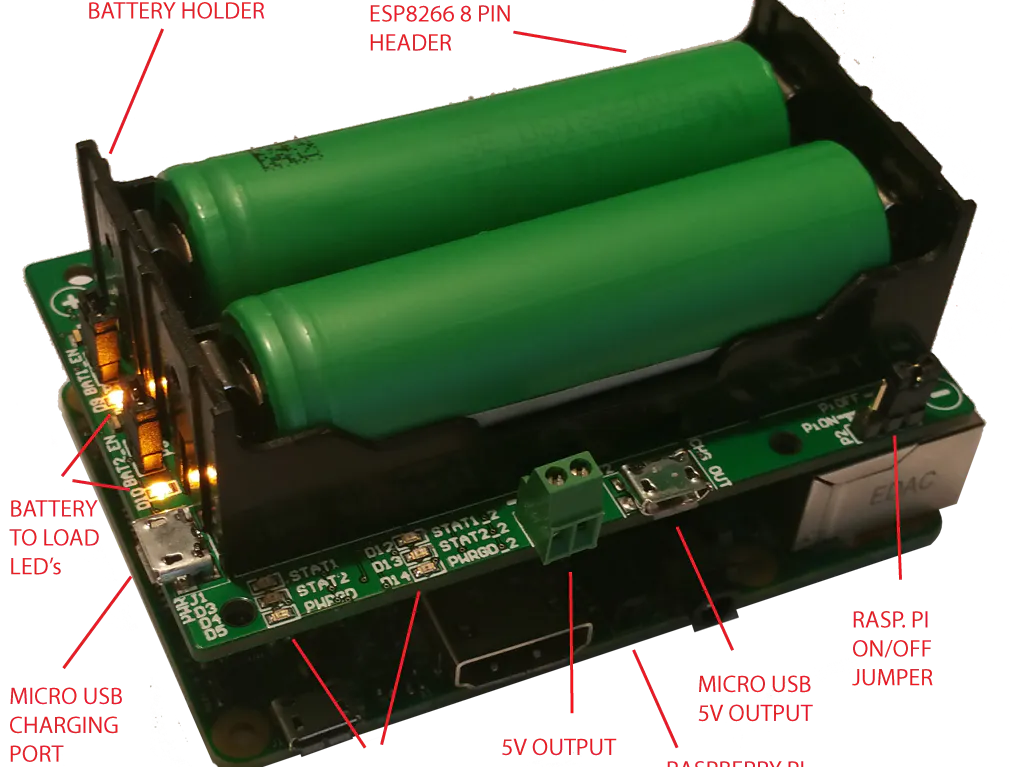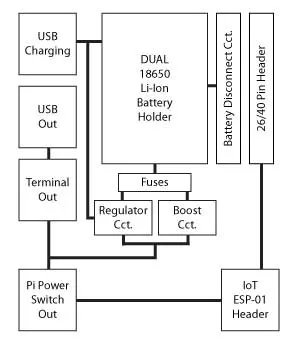Published

# Pi18650 DUAL LI-ION BATTERY HAT

Twice the power, Twice the capacity to power your Pi.

IntermediateFull instructions provided15 minutes4,588## Things used in this project

### Hardware components

 Pi18650 DUAL BATTERY HAT
×1
 18650 Lithium Ion Batteries
×2Raspberry Pi 3 Model B
×1

## Schematics

### Pi18650 Block Diagram## Code

### Dual Battery Monitoring Scipt

Python
This Python script monitors two Li-Ion batteries in the Pi18650 DUAL Battery HAT.
```import smbus
import time
from smbus import SMBus
import RPi.GPIO as GPIO

SAMPLES = 5.0
battery_capacity = 0.0
fuel_guage_temperature = 09.0
battery_voltage = 0.0
sample_delay = 0.005

BAT1_V_MON = 23
BAT2_V_MON = 24

GPIO.setmode(GPIO.BCM)
GPIO.setup(BAT1_V_MON, GPIO.OUT)
GPIO.setup(BAT2_V_MON, GPIO.OUT)

DEVICE_BUS = 1 #device bus number
DEVICE_REG_ADDR_ID = 0x00  #registry for device id ascii string 0x00 - 0x07

#Fuel Gas Guage Monitor Registers
FUELGUAGE_REG_ADDR_00 = 0X00	#status				(R) default()
FUELGUAGE_REG_ADDR_01 = 0X01	#control	 		(R/W) default(3C)
FUELGUAGE_REG_ADDR_02 = 0X02	#acc charge MSB 		(R/W) default(7F)
FUELGUAGE_REG_ADDR_03 = 0X03	#acc charte LSB 		(R/W) default(FF)
FUELGUAGE_REG_ADDR_04 = 0X04	#charge threshold high MSB 	(R/W) default(FF)
FUELGUAGE_REG_ADDR_05 = 0X05	#charge threshold high LSB 	(R/W) default(FF)
FUELGUAGE_REG_ADDR_06 = 0X06	#charge threshold low MSB 	(R/W) default(00)
FUELGUAGE_REG_ADDR_07 = 0X07	#charge threshold low LSB 	(R/W) default(00)
FUELGUAGE_REG_ADDR_08 = 0x08	#voltage MSB 			(R) default(XX) unknown
FUELGUAGE_REG_ADDR_09 = 0x09	#voltage LSB 			(R) default(XX)
FUELGUAGE_REG_ADDR_0A = 0X0A	#voltage threshold high 	(R/W) default(FF)
FUELGUAGE_REG_ADDR_0B = 0X0B	#voltage threshold low 		(R/W) default(00)
FUELGUAGE_REG_ADDR_0C = 0X0C	#temperature MSB 		(R) default(XX)
FUELGUAGE_REG_ADDR_0D = 0X0D	#temperature LSB 		(R) default(XX)
FUELGUAGE_REG_ADDR_0E = 0X0E	#temperature threshold high 	(R/W) default(FF)
FUELGUAGE_REG_ADDR_0F = 0X0F	#temperature threshold low 	(R/W) default(00)

bus = smbus.SMBus(DEVICE_BUS)

class i2cCommand:

def __init__(self):

global SAMPLES
global battery_capacity
global fuel_guage_temperature
global battery_voltage
global sample_delay

global BAT1_V_MON
global BAT2_V_MON

global bus

global FUELGUAGE_REG_ADDR_00 #= 0X00 #(reg name A)
global FUELGUAGE_REG_ADDR_01 #= 0X01 #(reg name B)
global FUELGUAGE_REG_ADDR_02 #= 0X02 #(reg name C)
global FUELGUAGE_REG_ADDR_03 #= 0X03 #(reg name D)
global FUELGUAGE_REG_ADDR_04 #= 0X04 #(reg name E)
global FUELGUAGE_REG_ADDR_05 #= 0X05 #(reg name F)
global FUELGUAGE_REG_ADDR_06 #= 0X06 #(reg name G)
global FUELGUAGE_REG_ADDR_07 #= 0X07 #(reg name H)
global FUELGUAGE_REG_ADDR_08 #= 0X08 #(reg name I)
global FUELGUAGE_REG_ADDR_09 #= 0X09 #(reg name J)
global FUELGUAGE_REG_ADDR_0A #= 0X0A #(reg name K)
global FUELGUAGE_REG_ADDR_0B #= 0X0B #(reg name L)
global FUELGUAGE_REG_ADDR_0C #= 0X0C #(reg name M)
global FUELGUAGE_REG_ADDR_0D #= 0X0D #(reg name N)
global FUELGUAGE_REG_ADDR_0E #= 0X0E #(reg name O)
global FUELGUAGE_REG_ADDR_0F #= 0X0F #(reg name P)

GPIO.output(BAT1_V_MON, False)	#set both n-chan mosfets to off for multiplexing I2C clk line
GPIO.output(BAT2_V_MON, False)

return None

def 	set_fuelguage_control_reg(self, adc_mode_bit7_6, prescaler_bit5_3, al_cc_bit2_1, shutdown_bit0):

#set bit7_6-11 (0xC0) for auto mode temp and volt convertion every 1 second
#bit7_6-10 (0x80) for manual volt mode
#bit7_6-01 (0x40) for manual temp mode
#bit7_6-00 (0x00) for sleep mode

#leave bit5_3-111 for prescaler default 128
#equation 2^(4*B5 + 2*B4 + B3) ie. 2^(4*1 + 2*1 + 1) = 2^7 = 128

#leave bit2_1-10 for output alert mode (can connect to pin on rasp pi compute module

#leave bit0-0 as the 3V3 power will be turned off by user, but current drain will not be monitored when 3V3 off

#hardcode for now
prescaler_bit5_3 = 0x38
al_cc_bit2_1 = 0x04
shutdown_bit0 = 0x00

value = adc_mode_bit7_6 | prescaler_bit5_3 | al_cc_bit2_1 | shutdown_bit0

print "set_fuel_gauage_control_reg value: ", hex(value)

for i in range(2):

if i == 0:
print("Initializing Battery 1 Monitor")
GPIO.output(BAT1_V_MON, GPIO.HIGH)
GPIO.output(BAT2_V_MON, GPIO.LOW)
else:
print("Initializing Battery 2 Monitory")
GPIO.output(BAT1_V_MON, GPIO.LOW)
GPIO.output(BAT2_V_MON, GPIO.HIGH)
try:

except IOError as e:

print("Could not connect to battery monitor: (set_fuelguage_control_reg) "), i

'''
print "checking fuel_gauage_control_reg value: "

print "ctrl reg: ", hex(device_str_ctrl)
'''

time.sleep(0.1)

return 0

def 	fuelguage_check_volt(self, battery_sel):

if battery_sel == 1:

GPIO.output(BAT1_V_MON, GPIO.HIGH)
GPIO.output(BAT2_V_MON, GPIO.LOW)

elif battery_sel == 2:

GPIO.output(BAT1_V_MON, GPIO.LOW)
GPIO.output(BAT2_V_MON, GPIO.HIGH)

else:

GPIO.output(BAT1_V_MON, GPIO.LOW)
GPIO.output(BAT2_V_MON, GPIO.LOW)

#voltage is 14bit (reg's I and J) and temperature 10bit (reg's M and N)resolution
#convert I and J to 16bit variable, then (value / 65535) x 6V = Voltage

#read registers I and J (8 and 9 sequentially)
voltage_msb = float(0.0)
voltage_lsb = float(0.0)
temp_msb = float(0.0)
temp_lsb = float(0.0)

#status, control, acc_msb, acc_lsb, chrgthhi_msb, chrgethhi_lsb, chrgthlow_msb, chrgthlow_lsb, voltage_msb, voltage_lsb, voltth_msb, voltth_lsb, temp_msb, temp_lsb, tempth_msb, tempth_lsb = all_regs
#print "all regs1: ", all_regs

battery1_status_flag = 0
battery2_status_flag = 0

try:

#print "voltage_msb: ", voltage_msb
#print "voltage_lsb: ", voltage_lsb

#print "temp_msb: ", temp_msb
#print "temp_lsb: ", temp_lsb

if battery_sel == 1:
battery1_status_flag = 1
else:
battery2_status_flag = 1

except IOError as e:

print("Could not read to battery voltage monitor: "), battery_sel

if battery_sel == 1:
battery1_status_flag = 0
else:
battery2_status_flag = 0

#print "voltage_reg_I_J: ", voltage_reg_I_J
#voltage_msb, voltage_lsb = voltage_reg_I_J

#print "voltage_msb: ", voltage_msb
#print "voltage_lsb: ", voltage_lsb

if (battery_sel == 1 and battery1_status_flag == 1) or (battery_sel == 2 and battery2_status_flag == 1):

voltage_16bit = float(0.0)
voltage_msb = voltage_msb << 8
#print "voltage_msb: ", hex(voltage_msb)
#print "voltage_lsb: ", hex(voltage_lsb)

voltage_16bit = float(voltage_msb | voltage_lsb)

#print "voltage_16bit: ", voltage_16bit

#voltage at battery
battery_volt = float(0.0)
divisor = float(65535.0)
multiplier = float(6.0)
battery_volt = ( voltage_16bit / divisor ) * multiplier

##print "battery_volt: ", battery_volt

battery_capacity_percent = 0

#battery capacity reference table
if battery_volt >= 4.20:
battery_capacity_percent = 100

elif  battery_volt < 4.20 and battery_volt >= 4.15:
battery_capacity_percent = 98

elif  battery_volt < 4.15 and battery_volt >= 4.10:
battery_capacity_percent = 95

elif  battery_volt < 4.10 and battery_volt >= 4.00:
battery_capacity_percent = 85

elif  battery_volt < 4.00 and battery_volt >= 3.95:
battery_capacity_percent = 75

elif  battery_volt < 3.95 and battery_volt >= 3.90:
battery_capacity_percent = 65

elif  battery_volt < 3.90 and battery_volt >= 3.85:
battery_capacity_percent = 55

elif  battery_volt < 3.85 and battery_volt >= 3.80:
battery_capacity_percent = 50

elif  battery_volt < 3.80 and battery_volt >= 3.75:
battery_capacity_percent = 48

elif  battery_volt < 3.75 and battery_volt >= 3.70:
battery_capacity_percent = 44

elif  battery_volt < 3.70 and battery_volt >= 3.65:
battery_capacity_percent = 38

elif  battery_volt < 3.65 and battery_volt >= 3.60:
battery_capacity_percent = 36

elif  battery_volt < 3.60 and battery_volt >= 3.55:
battery_capacity_percent = 34

elif  battery_volt < 3.55 and battery_volt >= 3.50:
battery_capacity_percent = 31

elif  battery_volt < 3.50 and battery_volt >= 3.45:
battery_capacity_percent = 29

elif  battery_volt < 3.45 and battery_volt >= 3.40:
battery_capacity_percent = 26

elif  battery_volt < 3.40 and battery_volt >= 3.35:
battery_capacity_percent = 24

elif  battery_volt < 3.35 and battery_volt >= 3.30:
battery_capacity_percent = 23

elif  battery_volt < 3.30 and battery_volt >= 3.25:
battery_capacity_percent = 21

elif  battery_volt < 3.25 and battery_volt >= 3.20:
battery_capacity_percent = 18

elif  battery_volt < 3.20 and battery_volt >= 3.15:
battery_capacity_percent = 16

elif  battery_volt < 3.15 and battery_volt >= 3.10:
battery_capacity_percent = 15

elif  battery_volt < 3.10 and battery_volt >= 3.05:
battery_capacity_percent = 11

elif  battery_volt < 3.05 and battery_volt >= 3.00:
battery_capacity_percent = 10
else:
battery_capacity_percent = 0

##print "battery_capacity_percent: ", battery_capacity_percent

#temperature conversion to celcius ------------------------------------------------

#print "temp_msb: ", temp_msb

#print "temp_lsb: ", temp_lsb

temperature_16bit = float(0.0)
temp_msb = temp_msb << 8

#print "temp_msb: ", hex(temp_msb)

temperature_16bit = float(temp_msb | temp_lsb)

#print "temperature_16bit: ", temperature_16bit

#voltage at battery
temperature_kelvin = float(0.0)
temperature_multiplier = float(600.0)
temperature_kelvin = ( temperature_16bit / divisor ) * temperature_multiplier

##print "temperature_kelvin: ", temperature_kelvin

temperature_celcius = float(0.0)

temperature_celcius = temperature_kelvin - 273.0

##print "temperature_celcius: ", temperature_celcius

elif (battery_sel == 1 and battery1_status_flag == 0) or (battery_sel == 2 and battery2_status_flag == 0):

print("No measurements available for battery: "), battery_sel
battery_volt = 0.0
battery_capacity_percent = 0
temperature_celcius = 0.0

return battery_capacity_percent, temperature_celcius, battery_volt

testpoll = i2cCommand()

testpoll.set_fuelguage_control_reg(0xC0, 0x80, 0x10, 0x00)

while 1:

battery_capacity = 0.0
fuel_guage_temperature = 0.0
battery_voltage = 0.0
battery_capacity_temp = 0.0
fuel_guage_temperature_temp = 0.0
battery_voltage_temp = 0.0

for i in range(int(SAMPLES)):

battery_temperature = testpoll.fuelguage_check_volt(1) #1 for battery 1,
battery_capacity_temp, fuel_guage_temperature_temp, battery_voltage_temp = battery_temperature
battery_capacity = battery_capacity + battery_capacity_temp
fuel_guage_temperature = fuel_guage_temperature + fuel_guage_temperature_temp
battery_voltage = battery_voltage + battery_voltage_temp
time.sleep(sample_delay)

battery_capacity = battery_capacity / SAMPLES
fuel_guage_temperature = fuel_guage_temperature / SAMPLES
battery_voltage = battery_voltage / SAMPLES

print "-----------------------------------------------"
print "Battery 1:"
print "Battery Capacity Percent: ", battery_capacity
print "Fuel Guage Temperature: ", fuel_guage_temperature
print "Battery Voltage: ", battery_voltage
time.sleep(2.0)
print "-----------------------------------------------"

battery_capacity = 0.0
fuel_guage_temperature = 0.0
battery_voltage = 0.0
battery_capacity_temp = 0.0
fuel_guage_temperature_temp = 0.0
battery_voltage_temp = 0.0

for i in range(int(SAMPLES)):

battery_temperature = testpoll.fuelguage_check_volt(2) #1 for battery 2,
battery_capacity_temp, fuel_guage_temperature_temp, battery_voltage_temp = battery_temperature
battery_capacity = battery_capacity + battery_capacity_temp
fuel_guage_temperature = fuel_guage_temperature + fuel_guage_temperature_temp
battery_voltage = battery_voltage + battery_voltage_temp
time.sleep(sample_delay)

battery_capacity = battery_capacity / SAMPLES
fuel_guage_temperature = fuel_guage_temperature / SAMPLES
battery_voltage = battery_voltage / SAMPLES

print "-----------------------------------------------"
print "Battery 2:"
print "Battery Capacity Percent: ", battery_capacity
print "Fuel Guage Temperature: ", fuel_guage_temperature
print "Battery Voltage: ", battery_voltage
time.sleep(2.0)
print "-----------------------------------------------"
print "*************************************************************************"
print "*************************************************************************"
```

## Credits

### Mark Chin

5 projects • 10 followers
Entrepreneur and Innovator of embedded systems, RFID and IoT.